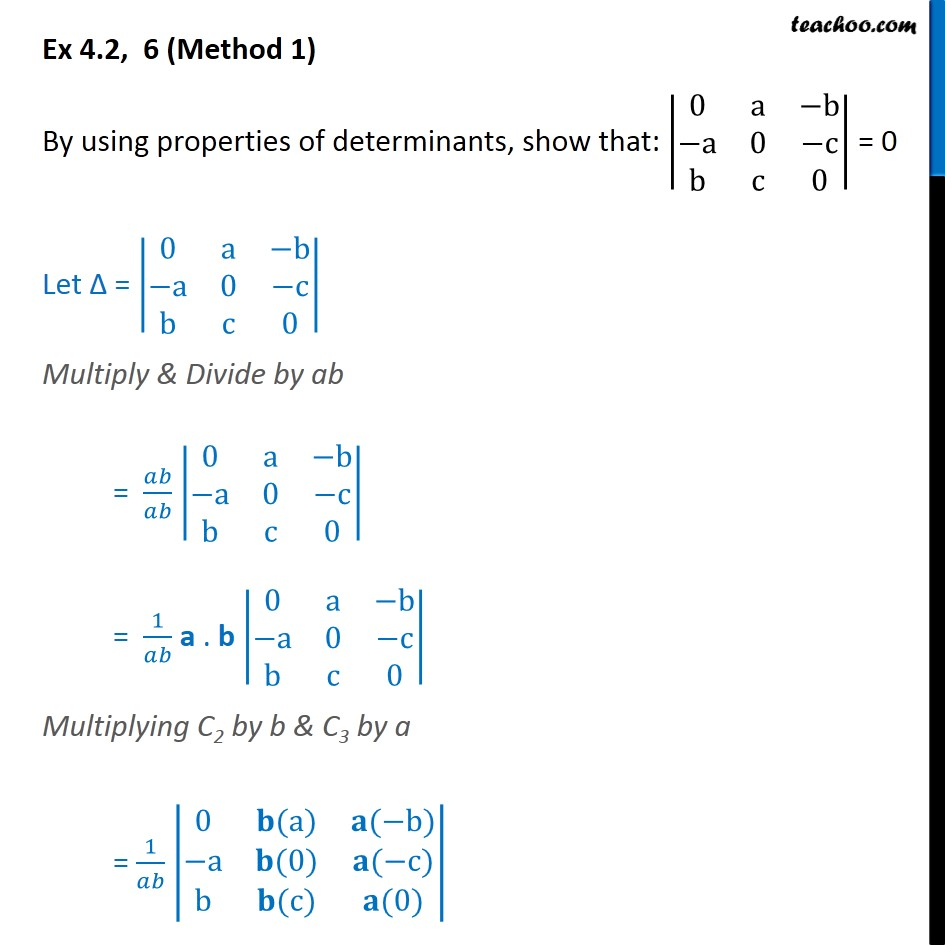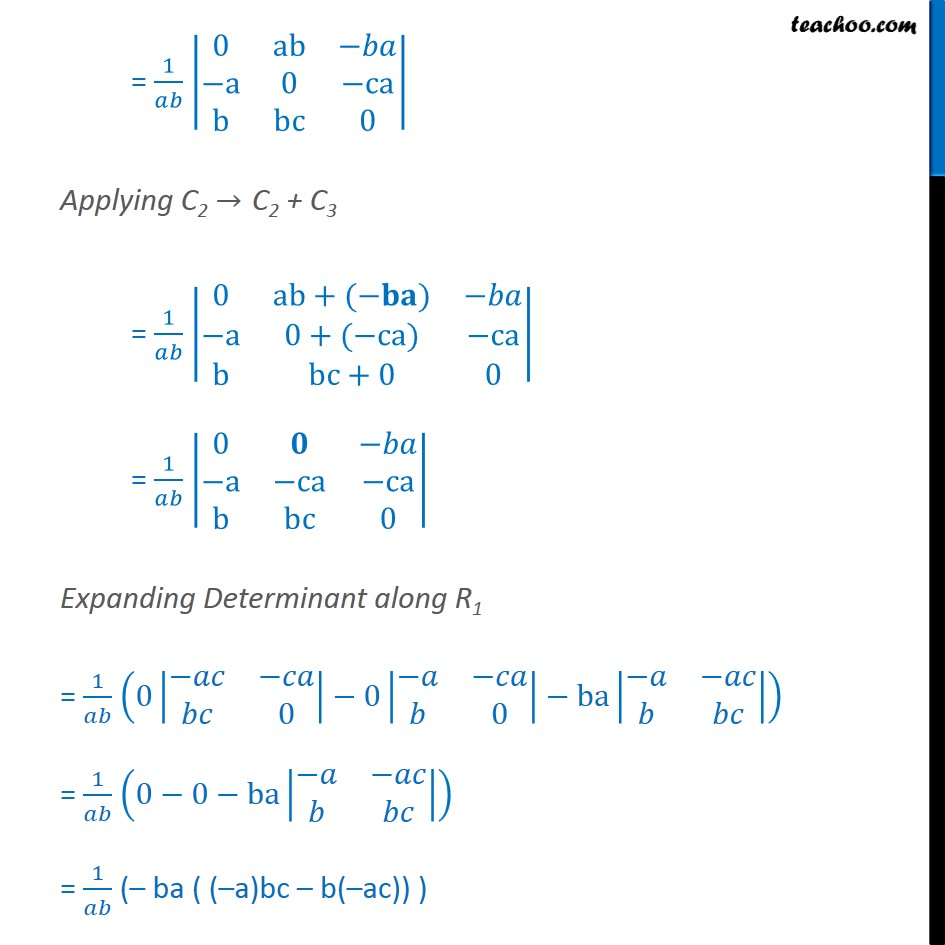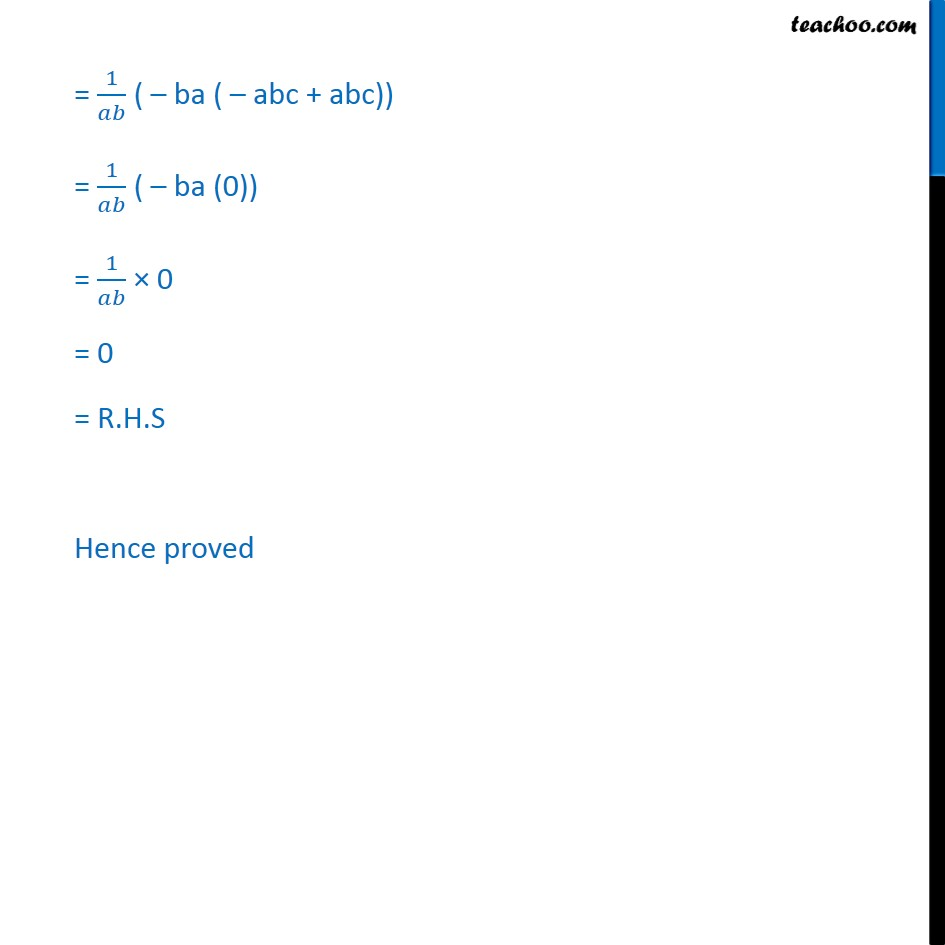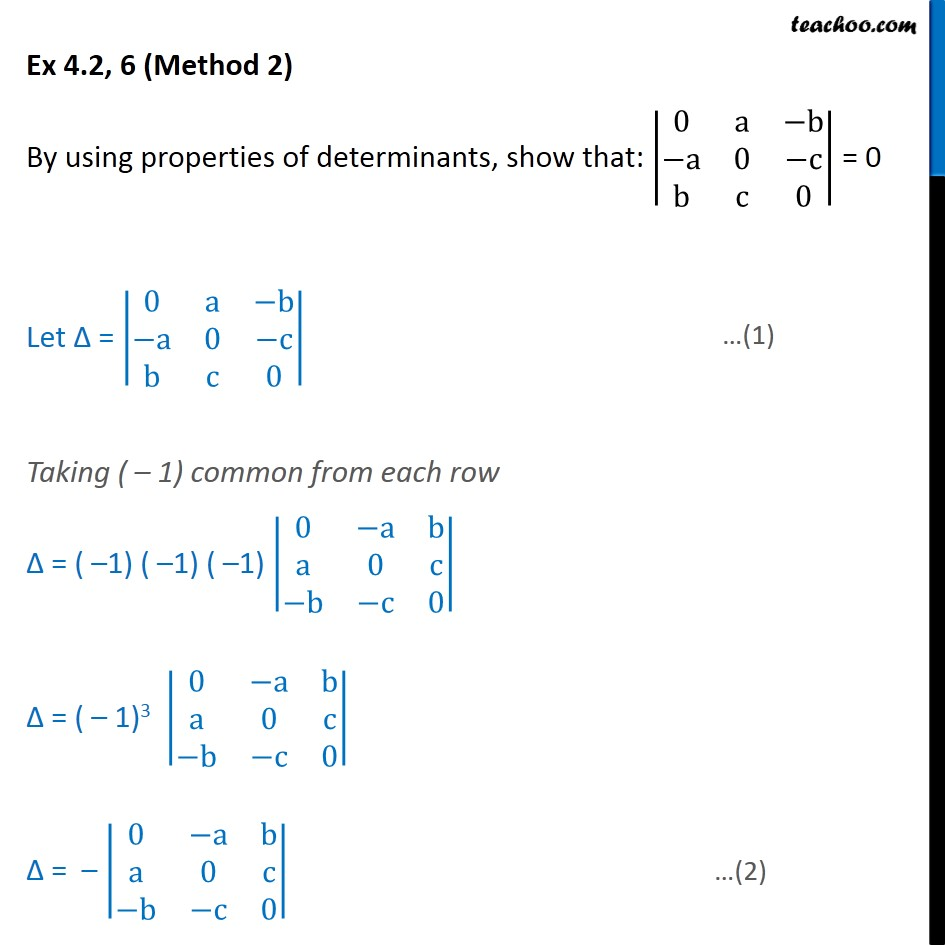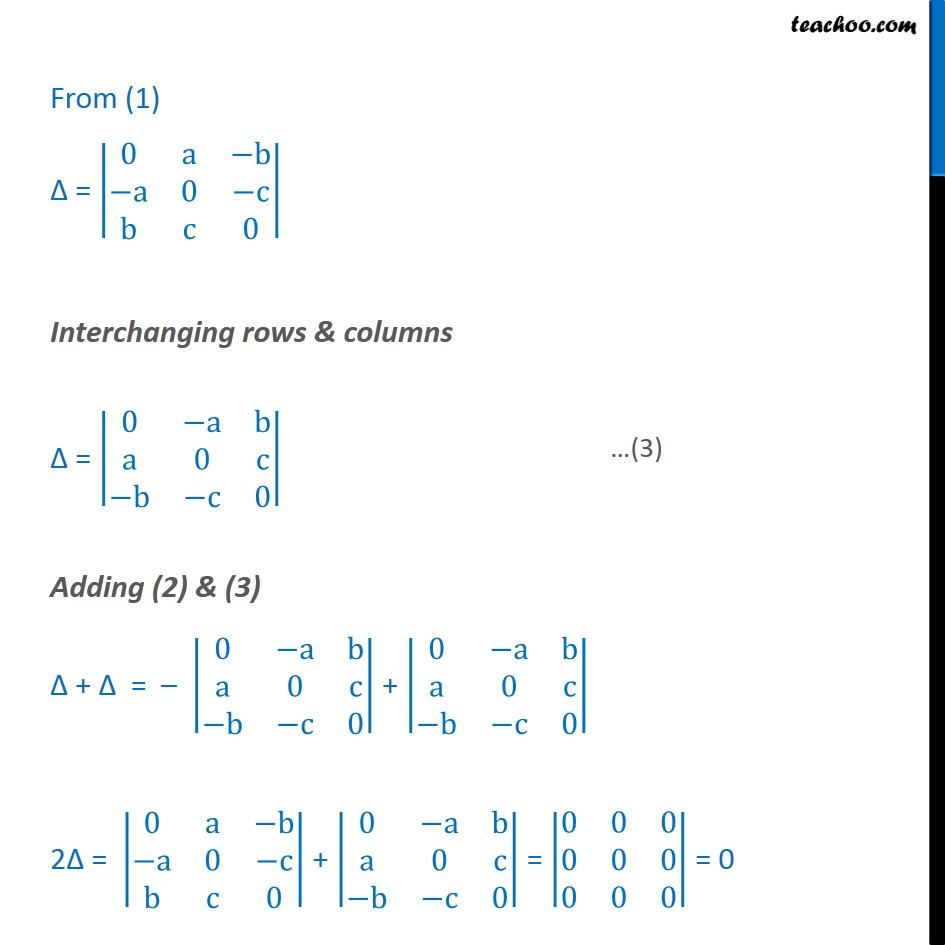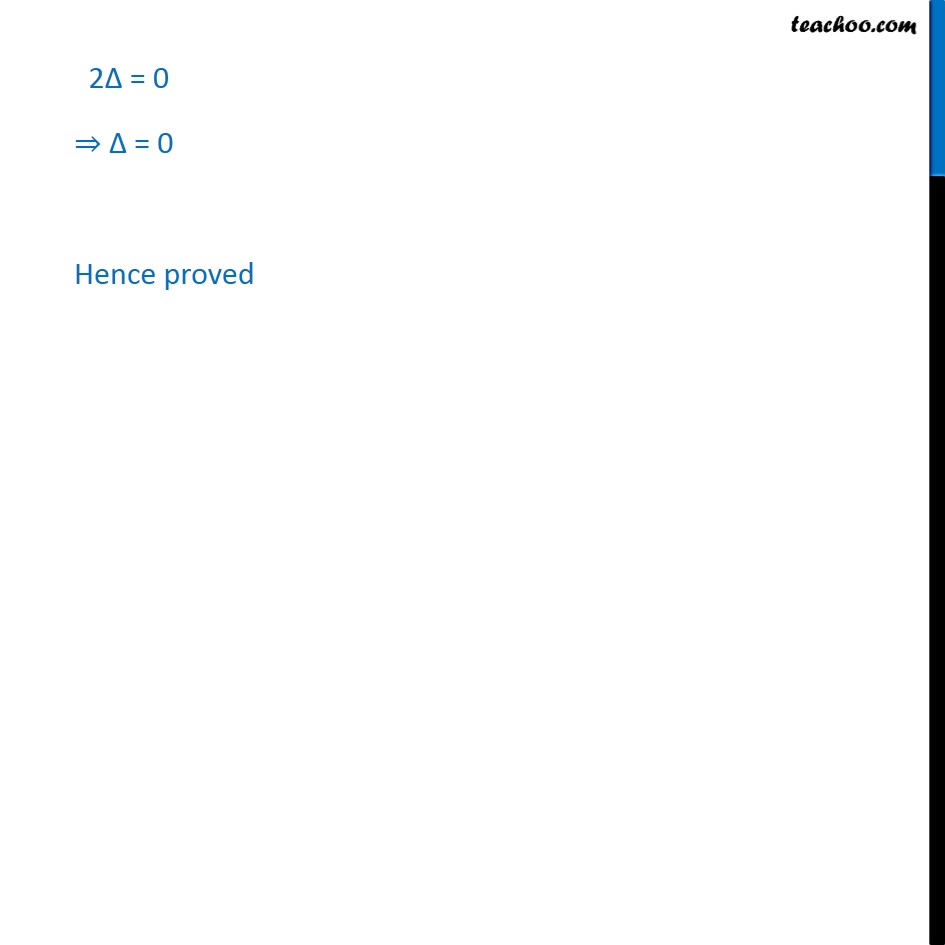1. Chapter 4 Class 12 Determinants
2. Serial order wise
3. Ex 4.2

Transcript

Ex 4.2, 6 (Method 1) By using properties of determinants, show that: 0﷮a﷮−b﷮−a﷮0﷮−c﷮b﷮c﷮0﷯﷯ = 0 Let Δ = 0﷮a﷮−b﷮−a﷮0﷮−c﷮b﷮c﷮0﷯﷯ Multiply & Divide by ab = 𝑎𝑏﷮𝑎𝑏﷯ 0﷮a﷮−b﷮−a﷮0﷮−c﷮b﷮c﷮0﷯﷯ = 1﷮𝑎𝑏﷯ a . b 0﷮a﷮−b﷮−a﷮0﷮−c﷮b﷮c﷮0﷯﷯ Multiplying C2 by b & C3 by a = 1﷮𝑎𝑏﷯ 0﷮𝐛(a)﷮𝐚(−b)﷮−a﷮𝐛(0)﷮𝐚(−c)﷮b﷮𝐛(c)﷮𝐚(0)﷯﷯ = 1﷮𝑎𝑏﷯ 0﷮ab﷮−𝑏𝑎﷮−a﷮0﷮−ca﷮b﷮bc﷮0﷯﷯ Applying C2 → C2 + C3 = 1﷮𝑎𝑏﷯ 0﷮ab+(−𝐛𝐚)﷮−𝑏𝑎﷮−a﷮0+(−ca)﷮−ca﷮b﷮bc+0﷮0﷯﷯ = 1﷮𝑎𝑏﷯ 0﷮𝟎﷮−𝑏𝑎﷮−a﷮−ca﷮−ca﷮b﷮bc﷮0﷯﷯ Expanding Determinant along R1 = 1﷮𝑎𝑏﷯ 0 −𝑎𝑐﷮−𝑐𝑎﷮𝑏𝑐﷮0﷯﷯−0 −𝑎﷮−𝑐𝑎﷮𝑏﷮0﷯﷯−ba −𝑎﷮−𝑎𝑐﷮𝑏﷮𝑏𝑐﷯﷯﷯ = 1﷮𝑎𝑏﷯ 0−0−ba −𝑎﷮−𝑎𝑐﷮𝑏﷮𝑏𝑐﷯﷯﷯ = 1﷮𝑎𝑏﷯ (– ba ( (–a)bc – b(–ac)) ) = 1﷮𝑎𝑏﷯ ( – ba ( – abc + abc)) = 1﷮𝑎𝑏﷯ ( – ba (0)) = 1﷮𝑎𝑏﷯ × 0 = 0 = R.H.S Hence proved Ex 4.2, 6 (Method 2) By using properties of determinants, show that: 0﷮a﷮−b﷮−a﷮0﷮−c﷮b﷮c﷮0﷯﷯ = 0 Let Δ = 0﷮a﷮−b﷮−a﷮0﷮−c﷮b﷮c﷮0﷯﷯ Taking ( – 1) common from each row Δ = ( –1) ( –1) ( –1) 0﷮−a﷮b﷮a﷮0﷮c﷮−b﷮−c﷮0﷯﷯ Δ = ( – 1)3 0﷮−a﷮b﷮a﷮0﷮c﷮−b﷮−c﷮0﷯﷯ Δ = – 0﷮−a﷮b﷮a﷮0﷮c﷮−b﷮−c﷮0﷯﷯ From (1) Δ = 0﷮a﷮−b﷮−a﷮0﷮−c﷮b﷮c﷮0﷯﷯ Interchanging rows & columns Δ = 0﷮−a﷮b﷮a﷮0﷮c﷮−b﷮−c﷮0﷯﷯ Adding (2) & (3) Δ + ∆ = – 0﷮−a﷮b﷮a﷮0﷮c﷮−b﷮−c﷮0﷯﷯ + 0﷮−a﷮b﷮a﷮0﷮c﷮−b﷮−c﷮0﷯﷯ 2∆ = 0﷮a﷮−b﷮−a﷮0﷮−c﷮b﷮c﷮0﷯﷯ + 0﷮−a﷮b﷮a﷮0﷮c﷮−b﷮−c﷮0﷯﷯ = 0﷮0﷮0﷮0﷮0﷮0﷮0﷮0﷮0﷯﷯ = 0 2∆ = 0 ⇒ Δ = 0 Hence proved

Ex 4.2